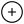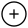# Popular Data Structure Operations Complexity Cheat Sheet

5 July, 2021

If you are someone who is Computer Science Student or Programmer, this list will be Handy to you. So don't forget to save it.

Data Structure Access Insertion Deletion
Array 1 n n
Stack n 1 1
Queue n 1 1
Hash Table - n n
Binary Search Tree n n n
B-Tree log(n) log(n) log(n)
Red-Black Tree log(n) log(n) log(n)
AVL Tree log(n) log(n) log(n)
Bloom Filter - 1 -

Data Structure Search Exceptional Cases
Array n
Stack n
Queue n
Hash Table n In the case of the perfect hash, function costs would be O(1)
Binary Search Tree n In the case of balanced tree, the costs would be of O(log(n))
B-Tree log(n)
Red-Black Tree log(n)
AVL Tree log(n)
Bloom Filter 1 False positives are possible while searching
Programming Programming competitivecoding coding computer science Data Structures
You need to be Logged in to Comment.# Popular Data Structure Operations Complexity Cheat Sheet

5 July, 2021

If you are someone who is Computer Science Student or Programmer, this list will be Handy to you. So don't forget to save it.

Data Structure Access Insertion Deletion
Array 1 n n
Stack n 1 1
Queue n 1 1
Hash Table - n n
Binary Search Tree n n n
B-Tree log(n) log(n) log(n)
Red-Black Tree log(n) log(n) log(n)
AVL Tree log(n) log(n) log(n)
Bloom Filter - 1 -

Data Structure Search Exceptional Cases
Array n
Stack n
Queue n
Hash Table n In the case of the perfect hash, function costs would be O(1)
Binary Search Tree n In the case of balanced tree, the costs would be of O(log(n))
B-Tree log(n)
Red-Black Tree log(n)
AVL Tree log(n)
Bloom Filter 1 False positives are possible while searching
Programming Programming competitivecoding coding computer science Data Structures